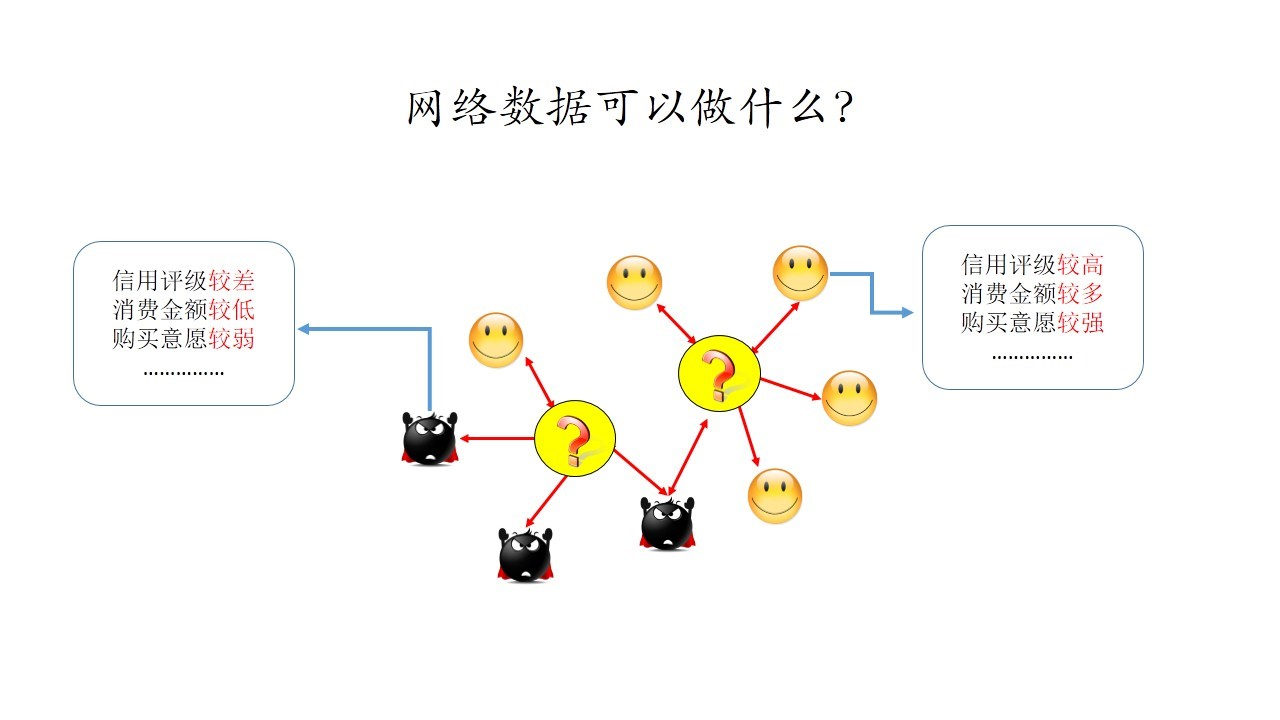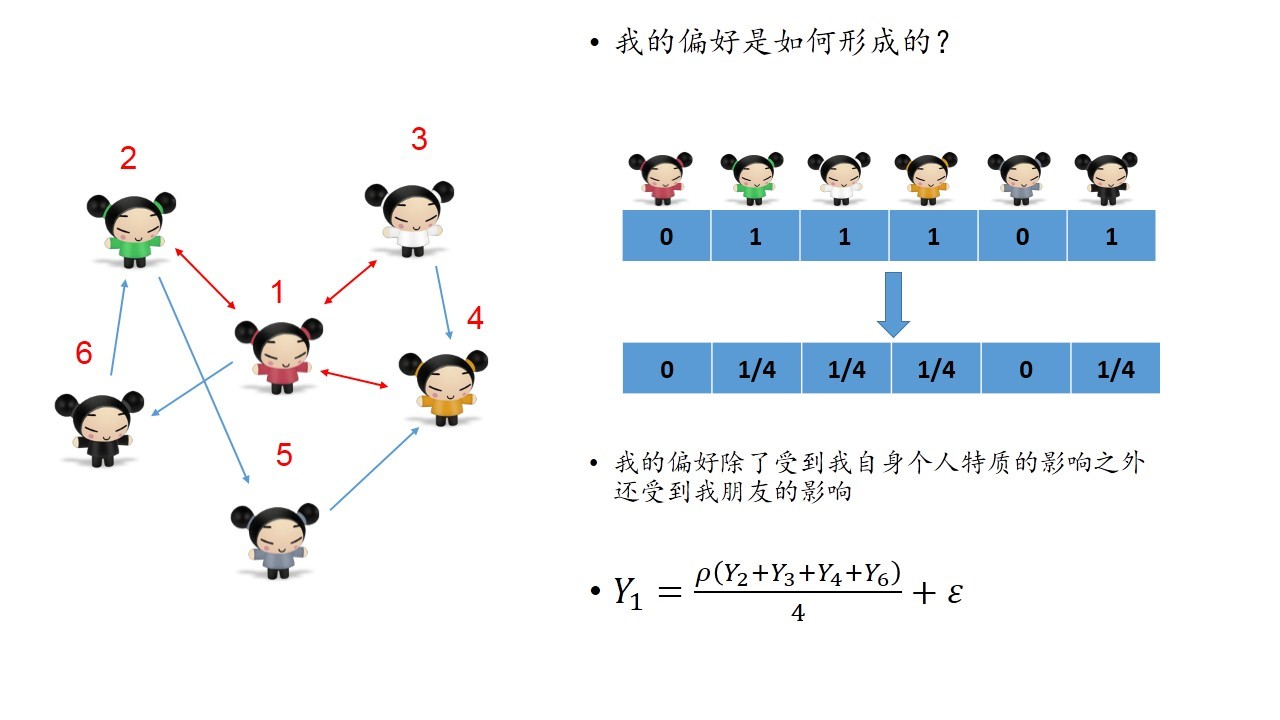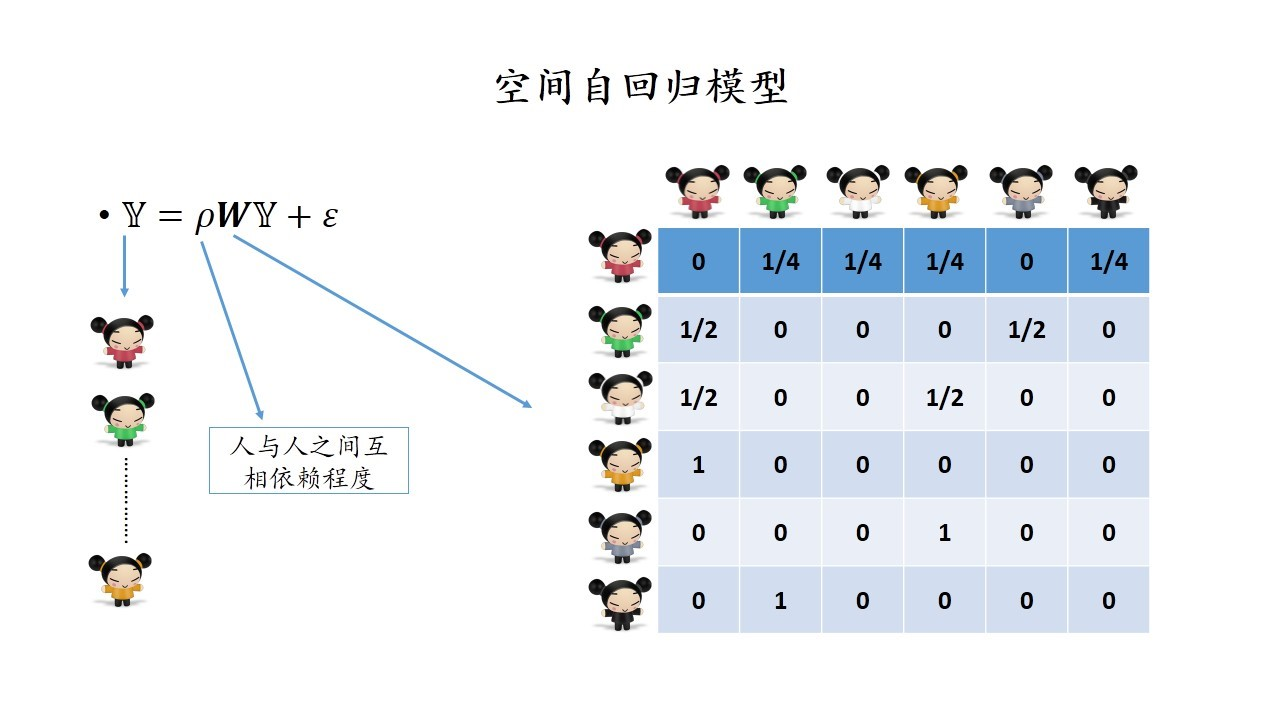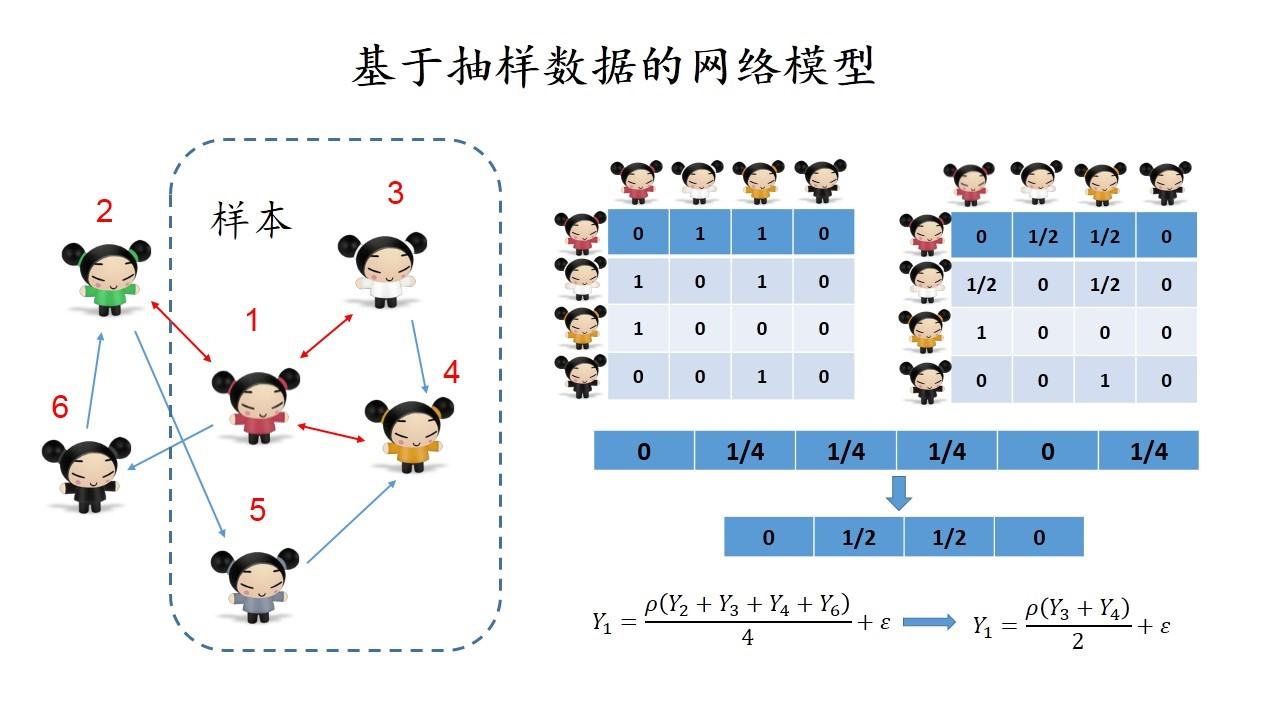# 一、音乐推荐

## 1.1 音乐推荐与社交网络Last.fm是 Audioscrobbler 音乐引擎设计团队的旗舰产品，有遍布232个国家超过1500万的活跃听众。2007年被CBS Interactive以2.8亿美元价格收购，目前，Last.fm是全球最大的社交音乐平台。QQ音乐是中国互联网领域领先的网络音乐平台及正版数字音乐服务提供商，在中国手机音乐市场所占份额跻身四大巨头（其他三个分别是酷狗、天天动听、酷我），月活跃用户已达到3亿，是中国社交音乐领域的领军人物。**以上事实说明音乐服务商在推荐算法上没有充分利用社交网络的信息。**事实上，在获得用户个人绑定社交网络账号的基础上，可以得到用户的朋友关系，进一步可以获得用户好友的听歌记录，这些歌曲可以成为初始推荐曲目的备选项，将这些备选项通过一定规则（热度、好友相似度）排序，可以用于音乐推荐；另外，众所周知，社交网络（如微博）是明星与粉丝互动的一个重要渠道，因此，可以重点提取用户对于社交网络中歌手以及音乐人的关注关系，以获得对用户偏好的推测。以上这些过程可以用下图表示。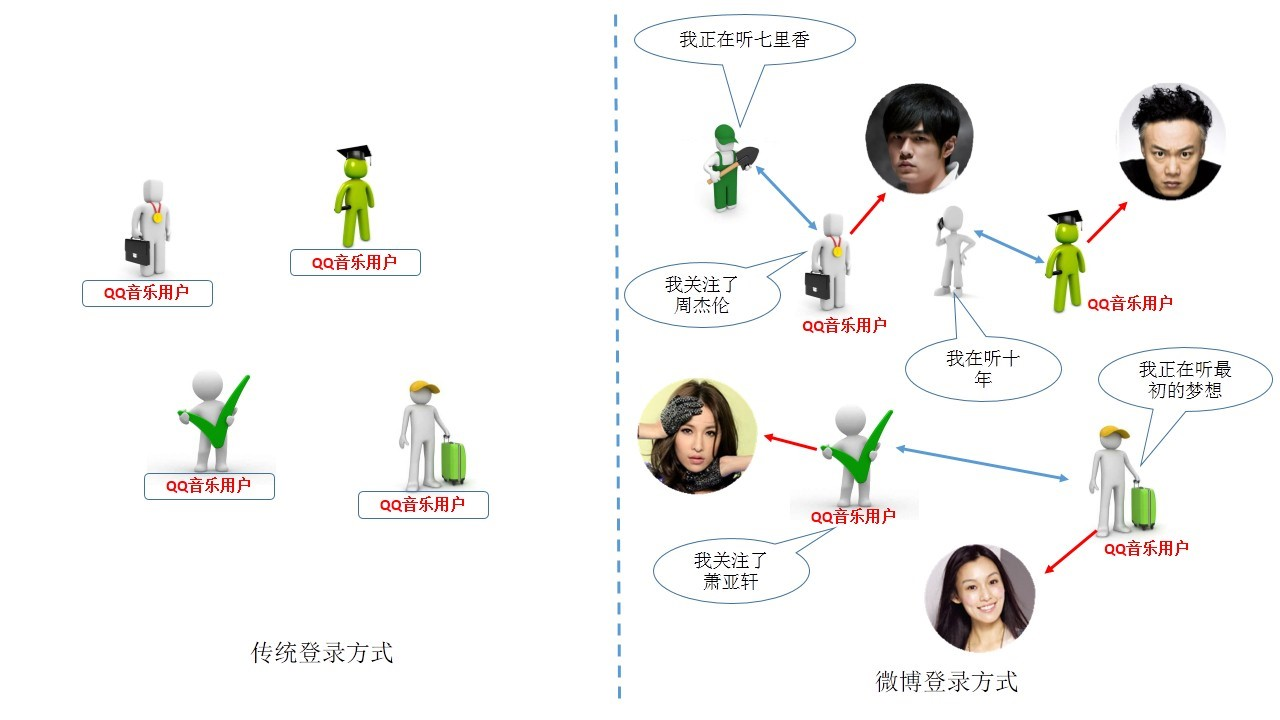## 1.2 基于社交网络的音乐推荐

### 用户信息整合

$$P(Y_{ik}=1)=f_{\theta_i}(X_{ik})$$

### 网络结构应用

$$p(Y_{ik}=1)=f_{\theta_i}(X_{ik},Y_{jk},Z_{qk}), \quad j \in \mathcal{N}_i ,q \in \mathcal{M}_i$$

# 二、新闻推荐

## 2.1 新闻推荐与社交网络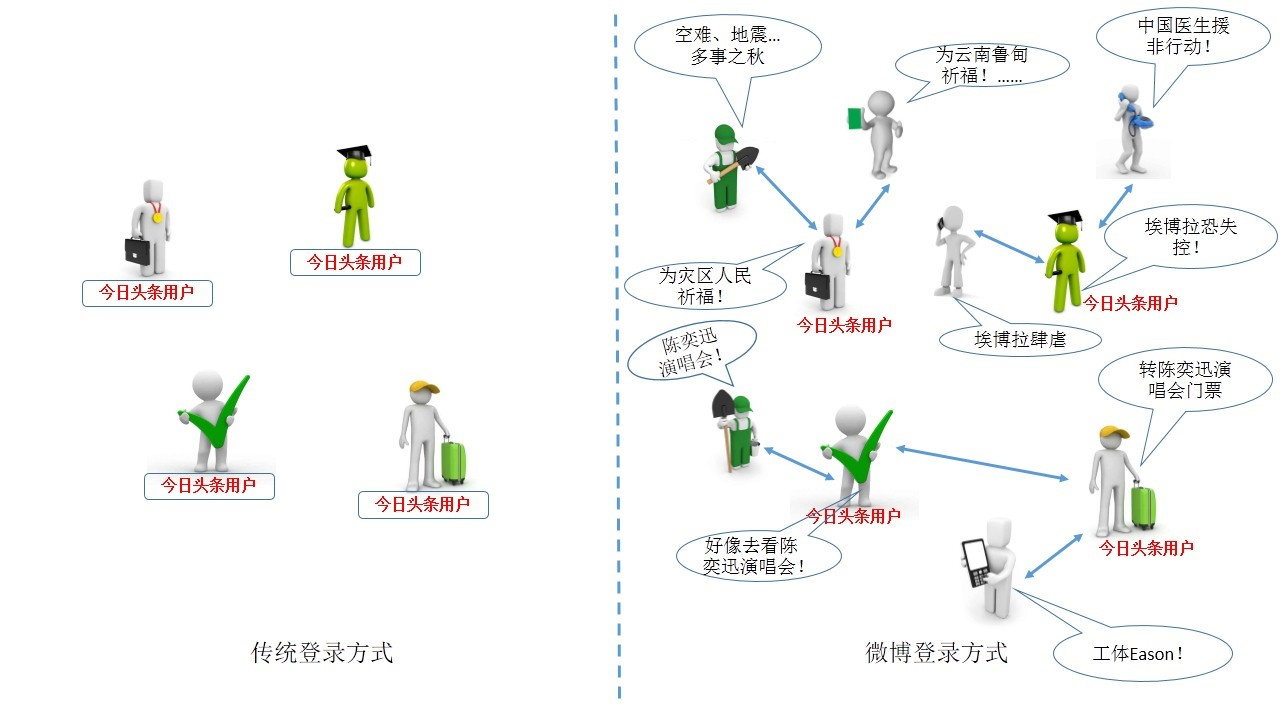2013年，张一鸣在接受采访时对今日头条的推荐系统构建进行了简述：今日头条会在用户绑定微博后的 5 秒钟之内为用户建立起一个 DNA 兴趣图谱。同时他也允许用户使用多社交网站的账号进行绑定，在个人动态中会滚动出现这些好友的一些信息动态。以上这些都是今日头条的优势所在，但是我们认为能真正的做好这几点今日头条仍然面临着一些挑战：①推荐的时候能否进行快速的线上计算是决定推荐速度的一个重要因素；如何进行多账号之间的数据融通和整合对充分挖掘好友关系并进行精准推荐具有重要作用；③如何应用好友对我的影响进行动态推荐信息中的排序是提高用户体验的另一个重要方式。总结以上优势和挑战，我们认为今日头条虽然是利用社交关系进行新闻推荐的先驱者，但是在利用网络数据的层面上仍有很大的改进和提升空间。

## 2.2 基于社交网络的新闻推荐

### 用户信息整合

$$p(Y_{ik}=1)=f_{\theta_i}(X_{ik})$$

### 网络结构应用

$$p(Y_{ik}=1)=f_{\theta_i}(X_{ik},X_{jk}) \quad j \in \mathcal{N}_i$$

# 三、 基于社交网络的其他应用

## 3.1 基于社交网络的个性化标签模型

### 3.1.1 社交网络中的个性化标签### 3.1.2 利用社交网络信息进行标签推断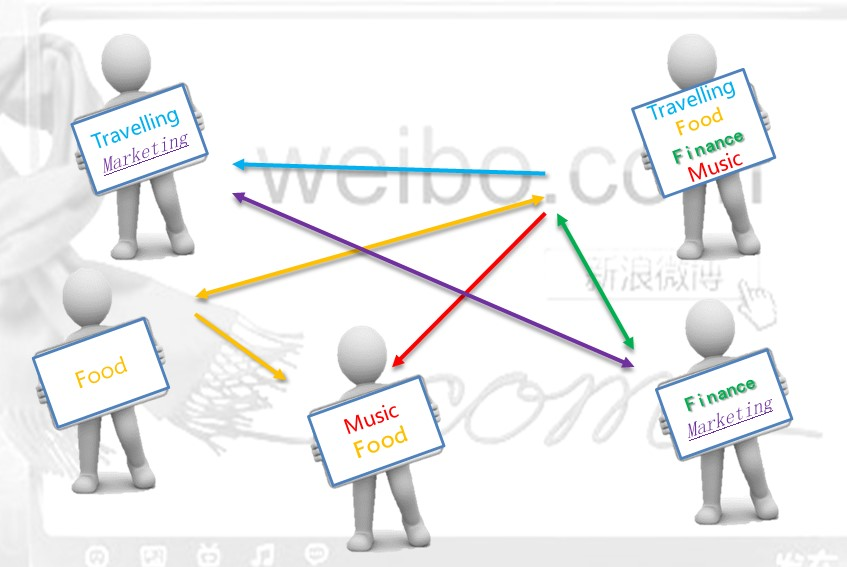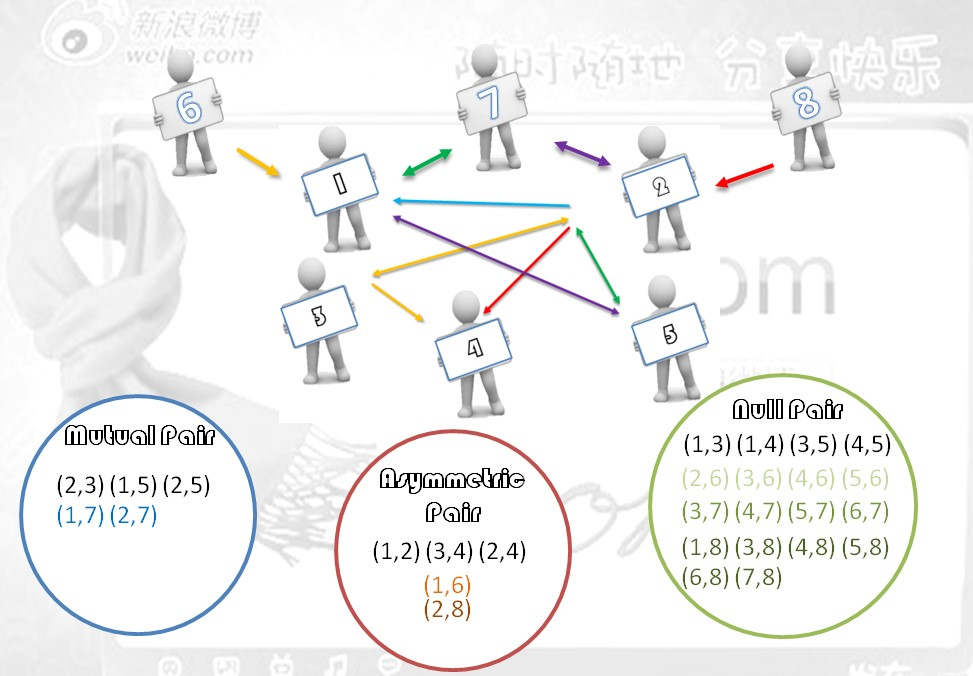### 3.1.3 实际案例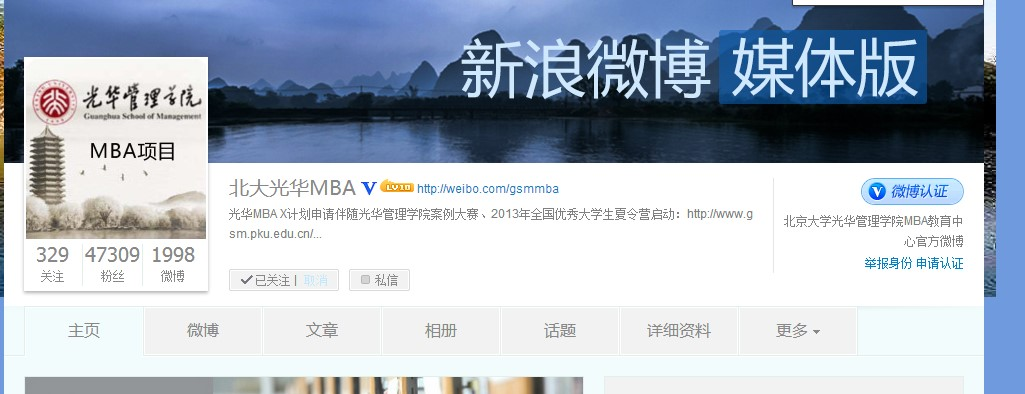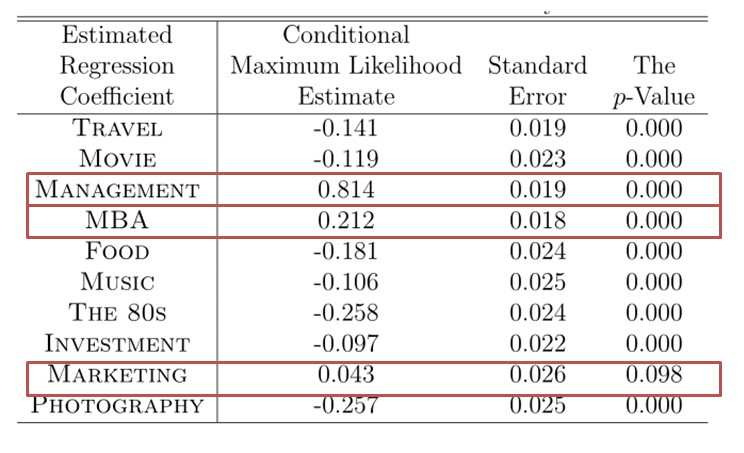## 3.2 基于社交网络评估个体影响力

### 3.2.1 网络影响力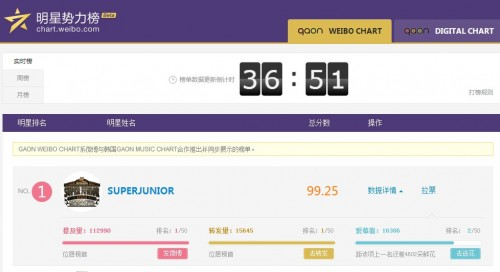### 3.2.2 评估网络影响力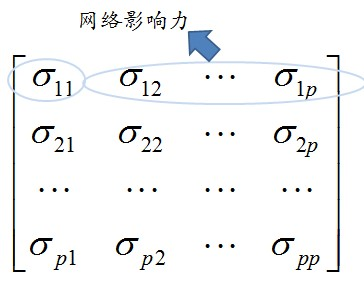$$\Sigma(A)=\beta_0I_p+\beta_1A+\cdots +\beta_dA^d$$

### 3.2.3 实际案例$$\Sigma(A)=1.17I_p +0.12A+0.07A^2$$

## 3.3 基于网络结构数据的客户关系管理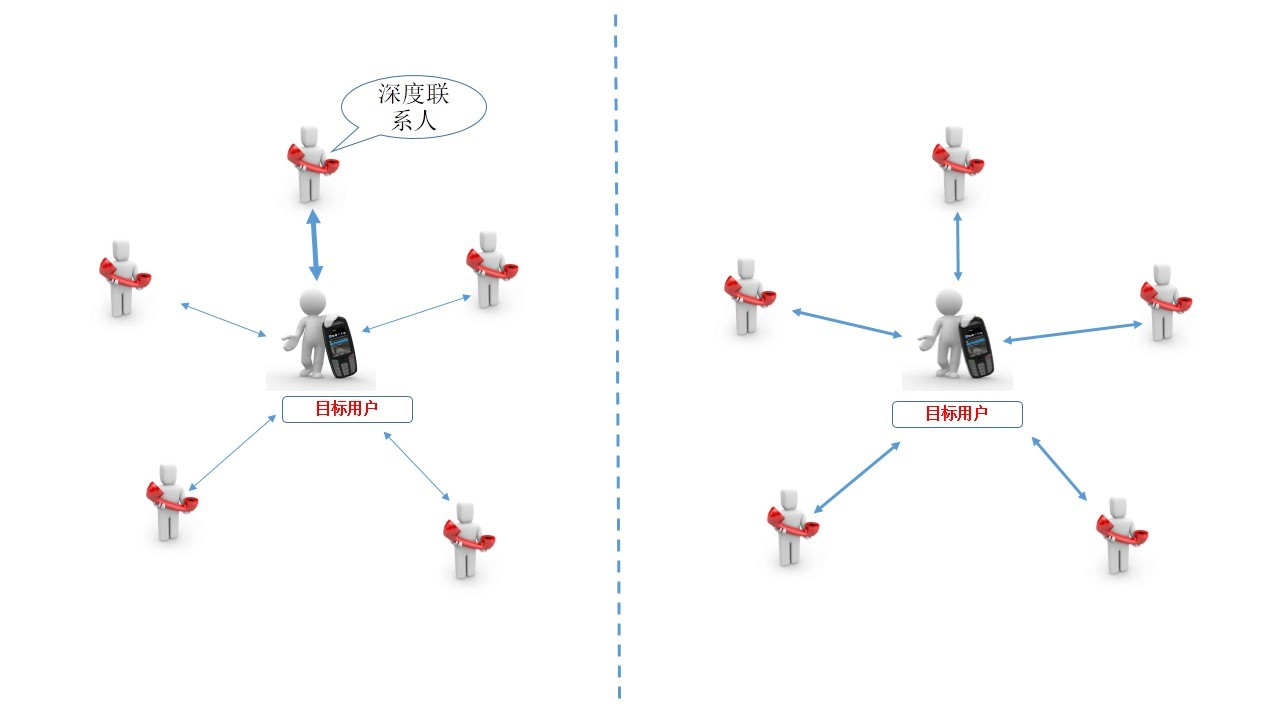## 3.4 网络结构数据的抽样与计算